Define PDE VI

LabVIEW 2018 Help

Edition Date: March 2018
Part Number: 371361R-01
View Product InfoLabVIEW 2016 HelpLabVIEW 2017 HelpLabVIEW 2018 HelpLabVIEW 2019 HelpLabVIEW 2020 Help

Owning Palette: Partial Differential Equations VIs

Requires: Full Development System

Defines the right side of a partial differential equation and its coefficients. You must manually select the polymorphic instance to use.

Use the pull-down menu to select an instance of this VI.

 Select an instance 2D Helmholtz (F(x, y) is Numeric)2D Helmholtz (F(x, y) is VI)1D Heat (F(t, x) is Numeric)1D Heat (F(t, x) is VI)2D Heat (F(t, x, y) is Numeric)2D Heat (F(t, x, y) is VI)1D Wave (F(t, x) is Numeric)1D Wave (F(t, x) is VI)2D Wave (F(t, x, y) is Numeric)2D Wave (F(t, x, y) is VI)

2D Helmholtz (F(x, y) is Numeric)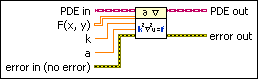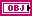PDE in is the class that stores the data of the equation.F(x, y) specifies the value of the right side of the equation. If the equation is defined on a rectangular domain, its size must be # of y-points-by-# of x-points from the Define PDE Domain VI. Each row or column of F(x, y) stores the values of the right side of the function evaluated on a particular y- or x-point. If the equation is defined on a polygonal domain, the number of columns must be equal to the number of Grid Points from the Define PDE Domain VI. LabVIEW uses only the first row of F(x, y), which stores the values of the right side of the function evaluated on Grid Points. By default, LabVIEW assumes the values of F(x, y) are zeros.k is a squared value that specifies the coefficient of the second order partial derivative of the unknown function in the equation. k cannot be 0. The default is 1.a specifies the coefficient of the unknown function in the equation. The default is 0.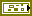error in describes error conditions that occur before this node runs. This input provides standard error in functionality.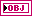PDE out returns the right side of PDE in and its coefficients.error out contains error information. This output provides standard error out functionality.

2D Helmholtz (F(x, y) is VI)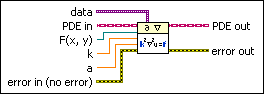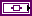data is a variant that passes arbitrary values to the F(x, y) VI.PDE in is the class that stores the data of the equation.F(x, y) is a strictly typed reference to the VI that implements the right side of the equation. Create this VI by starting from the VI template located in labview\vi.lib\gmath\pde.llb\Common\2D Stationary PDE Func Template.vit.k is a squared value that specifies the coefficient of the second order partial derivative of the unknown function in the equation. k cannot be 0. The default is 1.a specifies the coefficient of the unknown function in the equation. The default is 0.error in describes error conditions that occur before this node runs. This input provides standard error in functionality.PDE out returns the right side of PDE in and its coefficients.error out contains error information. This output provides standard error out functionality.

1D Heat (F(t, x) is Numeric)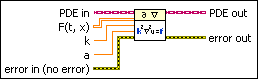PDE in is the class that stores the data of the equation.F(t, x) specifies the value of right side of the equation. The size of the right side of the equation must be # of t-points-by-# of x-points from the Define PDE Domain VI. Each row or column of F(t, x) stores the value of the right side of the equation evaluated on point X from the Define PDE Domain VI at a particular time step. By default, LabVIEW assumes the values of F(t, x) are zeros.k is a squared value that specifies the coefficient of the second order partial derivative of the unknown function in the equation. k cannot be 0. The default is 1.a specifies the coefficient of the unknown function in the equation. The default is 0.error in describes error conditions that occur before this node runs. This input provides standard error in functionality.PDE out returns the right side of PDE in and its coefficients.error out contains error information. This output provides standard error out functionality.

1D Heat (F(t, x) is VI)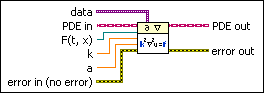data is a variant that passes arbitrary values to the F(t, x) VI.PDE in is the class that stores the data of the equation.F(t, x) is a strictly typed reference to the VI that implements the right side of the function. Create this VI by starting from the VI template located in labview\vi.lib\gmath\pde.llb\Common\1D Evolutionary PDE Func Template.vit.k is a squared value that specifies the coefficient of the second order partial derivative of the unknown function in the equation. k cannot be 0. The default is 1.a specifies the coefficient of the unknown function in the equation. The default is 0.error in describes error conditions that occur before this node runs. This input provides standard error in functionality.PDE out returns the right side of PDE in and its coefficients.error out contains error information. This output provides standard error out functionality.

2D Heat (F(t, x, y) is Numeric)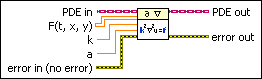PDE in is the class that stores the data of the equation.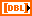F(t, x, y) specifies the value of the right side of the equation. The size of the right side of the equation must be # of t-points-by-# of y-points-by-# of x-points from the Define PDE Domain VI. Each page of F(t, x, y) stores the value of the right side of the equation evaluated on mesh grid points (X, Y) from the Define PDE Domain VI at a particular time step. On one page of F(t, x, y), each row or column stores the values of the right side of the equation evaluated on a particular y- or x-point. By default, LabVIEW assumes the values of F(t, x, y) are zeros.k is a squared value that specifies the coefficient of the second order partial derivative of the unknown function in the equation. k cannot be 0. The default is 1.a specifies the coefficient of the unknown function in the equation. The default is 0.error in describes error conditions that occur before this node runs. This input provides standard error in functionality.PDE out returns the right side of PDE in and its coefficients.error out contains error information. This output provides standard error out functionality.

2D Heat (F(t, x, y) is VI)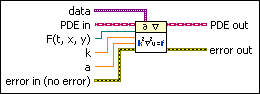data is a variant that passes arbitrary values to the F(t, x, y) VI.PDE in is the class that stores the data of the equation.F(t, x, y) is a strictly typed reference to the VI that implements the right side of the equation. Create this VI by starting from the VI template located in labview\vi.lib\gmath\pde.llb\Common\2D Evolutionary PDE Func Template.vit.k is a squared value that specifies the coefficient of the second order partial derivative of the unknown function in the equation. k cannot be 0. The default is 1.a specifies the coefficient of the unknown function in the equation. The default is 0.error in describes error conditions that occur before this node runs. This input provides standard error in functionality.PDE out returns the right side of PDE in and its coefficients.error out contains error information. This output provides standard error out functionality.

1D Wave (F(t, x) is Numeric)PDE in is the class that stores the data of the equation.F(t, x) specifies the value of right side of the equation. The size of the right side of the equation must be # of t-points-by-# of x-points from the Define PDE Domain VI. Each row or column of F(t, x) stores the value of the right side of the equation evaluated on point X from the Define PDE Domain VI at a particular time step. By default, LabVIEW assumes the values of F(t, x) are zeros.k is a squared value that specifies the coefficient of the second order partial derivative of the unknown function in the equation. k cannot be 0. The default is 1.a specifies the coefficient of the unknown function in the equation. The default is 0.error in describes error conditions that occur before this node runs. This input provides standard error in functionality.PDE out returns the right side of PDE in and its coefficients.error out contains error information. This output provides standard error out functionality.

1D Wave (F(t, x) is VI)data is a variant that passes arbitrary values to the VI.PDE in is the class that stores the data of the equation.F(t, x) is a strictly typed reference to the VI that implements the right side of the function. Create this VI by starting from the VI template located in labview\vi.lib\gmath\pde.llb\Common\1D Evolutionary PDE Func Template.vit.k is a squared value that specifies the coefficient of the second order partial derivative of the unknown function in the equation. k cannot be 0. The default is 1.a specifies the coefficient of the unknown function in the equation. The default is 0.error in describes error conditions that occur before this node runs. This input provides standard error in functionality.PDE out returns the right side of PDE in and its coefficients.error out contains error information. This output provides standard error out functionality.

2D Wave (F(t, x, y) is Numeric)PDE in is the class that stores the data of the equation.F(t, x, y) specifies the value of the right side of the equation. The size of the right side of the equation must be # of t-points-by-# of y-points-by-# of x-points from the Define PDE Domain VI. Each page of F(t, x, y) stores the value of the right side of the equation evaluated on mesh grid points (X, Y) from the Define PDE Domain VI at a particular time step. On one page of F(t, x, y), each row or column stores the values of the right side of the equation evaluated on a particular y- or x-point. By default, LabVIEW assumes the values of F(t, x, y) are zeros.k is a squared value that specifies the coefficient of the second order partial derivative of the unknown function in the equation. k cannot be 0. The default is 1.a specifies the coefficient of the unknown function in the equation. The default is 0.error in describes error conditions that occur before this node runs. This input provides standard error in functionality.PDE out returns the right side of PDE in and its coefficients.error out contains error information. This output provides standard error out functionality.

2D Wave (F(t, x, y) is VI)data is a variant that passes arbitrary values to the F(t, x, y) VI.PDE in is the class that stores the data of the equation.F(t, x, y) is a strictly typed reference to the VI that implements the right side of the equation. Create this VI by starting from the VI template located in labview\vi.lib\gmath\pde.llb\Common\2D Evolutionary PDE Func Template.vit.k is a squared value that specifies the coefficient of the second order partial derivative of the unknown function in the equation. k cannot be 0. The default is 1.a specifies the coefficient of the unknown function in the equation. The default is 0.error in describes error conditions that occur before this node runs. This input provides standard error in functionality.PDE out returns the right side of PDE in and its coefficients.error out contains error information. This output provides standard error out functionality.

Define PDE Details

Helmholtz Equation

The following equation defines the Helmholtz equation: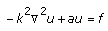where k and a are constant coefficients, u is the unknown function, and f is the right side of the equation. The operator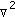is the Laplacian. The Laplacian in Cartesian coordinates is defined as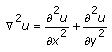in two-dimensional space and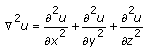in three-dimensional space.

Heat Equation

The following equation defines the general form of the heat equation: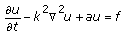Wave Equation

The following equation defines the general form of the wave equation: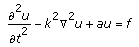Examples

Refer to the following VIs for examples of using the Define PDE VI:

• PDE Flexible Element VI: labview\examples\Mathematics\Differential Equations - PDE
• PDE String Vibration VI: labview\examples\Mathematics\Differential Equations - PDE
• PDE Thermal Distribution VI: labview\examples\Mathematics\Differential Equations - PDE